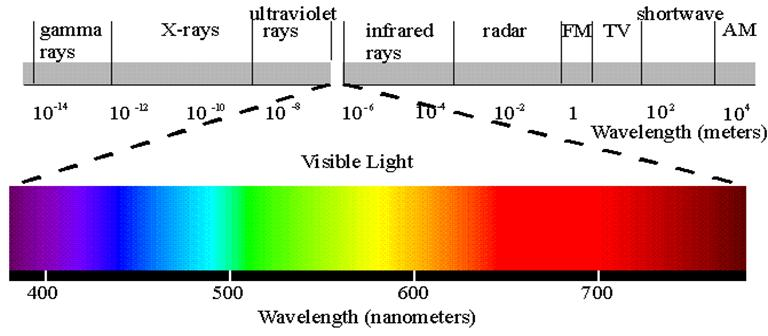How to verify the relationship between the wavelength and frequency of light

Law and Public Service High School, Manhattan

August 2006

1.  Aim/Problem

How to verify the relationship between the wavelength and frequency of light

2. Objectives

Physics -- Grades 9-12

Alignment with National Science Education Standards (National Academy Press, 2001):

·         To comprehend the relationship between the frequency and wavelength of light [Teaching Standard 9-12A –Teaching and assessment strategies, Development of student understanding]

·         Orally predict and physically verify the relationship between the frequency and wavelength of light [Content Standard 9-12A- Scientific inquiry]

·         Complete a design task as part of a structured cooperative team [Teaching Standard E- Nurture collaboration among students]

·         Create a setting for student work that is flexible and supportive of scientific inquiry [Teaching Standard D- Provide students with the time, space, and resources for learning science]

·         [Teaching Standard C- Engage in ongoing assessment of student learning]

·         [Teaching Standard E- Require students to take responsibility for the learning of all members of the community]

·         [Assessment Standard B- Achievement and opportunity to learn science must be assessed]

3. Background Knowledge

Students should have already learned:

·         The fundamental concepts of frequency (ƒ) and wavelength (λ) of light

·         Speed of light in vacuum, c= 3.00 x 108 m/s

·         C (m/s) = ƒ (Hz) λ (m)

4. Materials

Spectrometer

Fluorescent light (FL)

Calculator

Electromagnetic spectrum chart for different wavelengths

5. Procedure

Make groups of four heterogeneous students. Assign them the following roles: material manager, observer, recorder, and timekeeper.

·         Turn on the fluorescent light. Using a spectrometer look at the spectrum of fluorescent light. Determine the wavelength at the center of the bright band of each color, reading the scale to the nearest tenth.

·         Calculate the percentage error (P.E) for wavelength of each color by comparing calculated values to the original values that are given in the spectrum chart. Formula, P.E = original value (m) - calculated value (m)/ original value (m)

·         Record your observations in the following table:

 Color of fluorescent        light (FL) Spectrometer reading (near-est tenth) of wavelength (λ)  (nm) FL wavelength (λ) x 100 (nm) FL Wavelength (λ)  (m) Frequency (Hz) ƒ = c / λ Percentage error (P.E)  (m) Red Orange Yellow Green Blue Violet

6. Discussion and Assessment

Teacher will facilitate the the discussion by walking around and asking some key questions in the groups. For instance,

Q. Why does the calculated value differ from the original value?

Q. What happens to the frequency (ƒ) of light when wavelength (λ) decreases?

Q. Why do you see different colors of light?

7. Homework

Find out the frequency and corresponding wavelengths of the following electrical devices/signals that you use:

-Cell phone, I-pod, cable signal, Am and Fm radio

Note: This image is imported from the following web site only for educational purpose:

www.york.ca/eye /spectru.htm